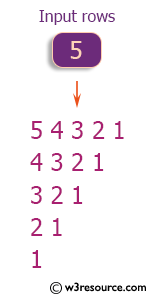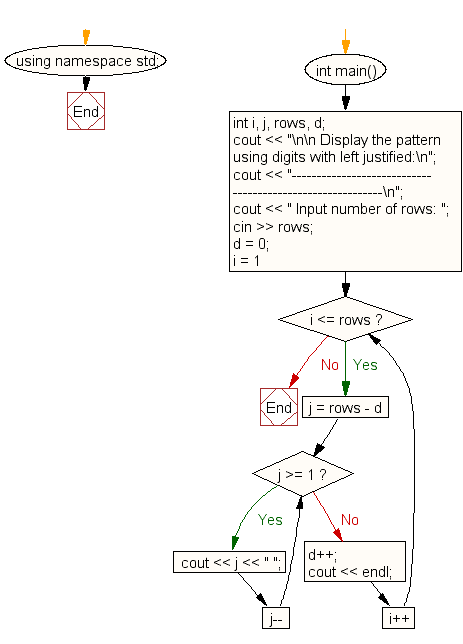﻿ C++ : Display the pattern using digits with left justified

# C++ Exercises: Display the pattern using digits with left justified and the highest columns appears in first row in descending order

## C++ For Loop: Exercise-52 with Solution

Write a C++ program to display the pattern using digits with left justified spacing and the highest columns appearing in the first row in descending order.

Pictorial Presentation:Sample Solution:

C++ Code :

``````#include <iostream>
using namespace std;

int main()
{
int i, j, rows, d;
cout << "\n\n Display the pattern using digits with left justified:\n";
cout << "----------------------------------------------------------\n";
cout << " Input number of rows: ";
cin >> rows;
d = 0;
for (i = 1; i <= rows; i++)
{
for (j = rows - d; j >= 1; j--)
{
cout << j << " ";
}
d++;
cout << endl;
}
}
``````

Sample Output:

``` Display the pattern using digits with left justified:
----------------------------------------------------------
Input number of rows: 5
5 4 3 2 1
4 3 2 1
3 2 1
2 1
1
```

Flowchart:C++ Code Editor: